# Electronics and Communication Engineering - Exam Questions Papers

6.

In a transmission line terminated with a load equal to the characteristic impedance, the reflection coefficient is

 A. 0 B. +1 C. -1 D. Infinity

Answer: Option A

Explanation: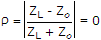.

7.

Consider a control system shown in given figure. For slight variation in G, the ratio of open loop sensitivity to closed loop sensitivity will be given by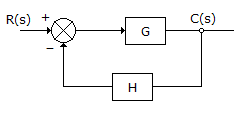A. 1 : (1 + GH) B. 1 : (1 + GH)- 1 C. 1 : (1 - GH) D. 1 : (1 - GH)- 1

Answer: Option B

Explanation:

Open loop sensitivity = 1

Closed loop sensitivity =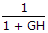Hence ratio = 1 : (1 + GH) - 1.

8.

Consider the signal S(t) shown below :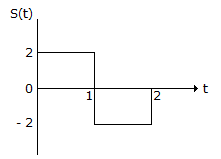The slope of the matched filter output during the interval

 A. 8 B. 12 C. 16 D. 18

Answer: Option A

Explanation:

Match filter will be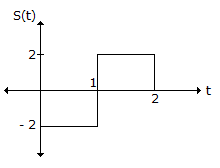The output of this will be convolution of S(t) with match filter.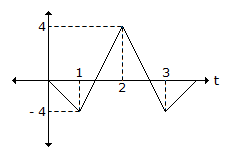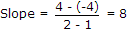.

9.

The logic function f(A B, C) = Σm(0, 2, 4, 5, 6) represented by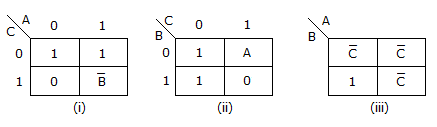A. i B. i, ii C. i, iii D. i, ii, iii

Answer: Option B

Explanation: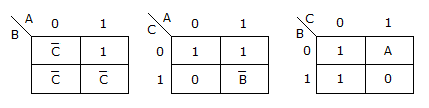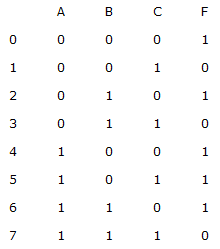10.

An electrical system and its signal-flow graph representation are shown in figure (a) and (b) respectively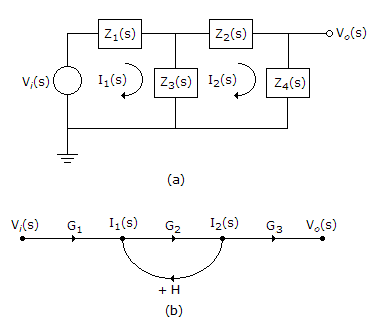The values of G2 and H, respectively are

 A.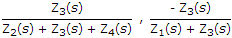B.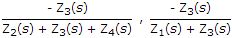C.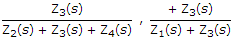D.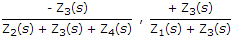Answer: Option A

Explanation:

Vi(s) = Z1I1 + Z3(I1 - I2) ...(i)

V0(s) = Z4I2

I2(Z3 + Z2 + Z4) = Z3I1 ...(ii)

From signal flow graph

I2 = I1G2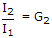Hence,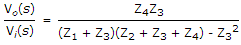...(iii)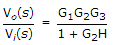From SFG ...(iv)

From (ii), (iii), (iv) we get H.

#### Current Affairs 2021

Interview Questions and Answers